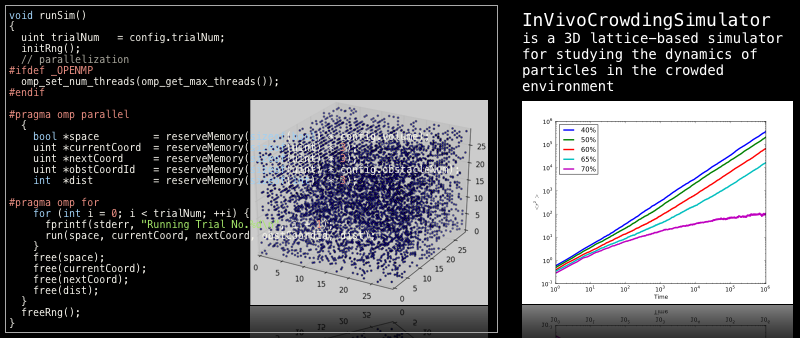### InVivoCrowdingSimulator: A 3D lattice-based simulator for studying the dynamics of particles in the crowded environment.

Latest release: 1.0.0, released 5th May 2012#### Overview

InVivoCrowdingSimulator (IVCSim) is a 3D lattice-based simulator for studying the dynamics of particles in the crowded environment (like in vivo environment). The simulator outputs MSD (Mean Square Displacement) corresponding to the time. The simulator uses "Blind Ant model" for Brownian motion. The library should build and work without serious troubles on Unix based operating systems (Linux, MacOSX and FreeBSD).

#### Dependencies

InVivoCrowdingSimulator (IVCSim) requires the GNU Scientific Library (GSL) which is a numerical library to be installed on your system. Please follow the instruction on GSL webpage and install GSL. If you have multi-core system (such as Core2Duo, Core2Quad, Core i5, Core i7, Phenome X4, etc.) and want to improve the performance of your simulation, compiling IVCSim with OpenMP might be your help.

### Installation

IVCSim is written in C99, thus it should build and work without serious troubles on Unix based operating systems (Linux, MacOSX and FreeBSD). We have tested IVCSim to work on Linux and MacOSX, both with and without OpenMP.

#### How to build IVCSim

1. Extract the archive file
2. ```tar xvzf invivosim-1.0.0.tar.gz
```
3. Compile
4. ```cd invivosim-1.0.0/
vim Makefile  (edit Makefile with your favorite editor)
```
modify CFLAGS and LDFLAGS to correctly find your GSL. By default, compiler will try to find the header files and libraries under /usr, /usr/local and /opt/local directories. This might work on most of the UNIX based operating systems. If your system have OpenMP, then you can enable it by uncommenting following 2 lines:
```	#OMPINC = -fopenmp
#OMPLIB = -lgomp
```
By using OpenMP, simulation will be automatically parallelized results to performance improvement. Finally, type 'make' and compilation will start.
```make
```

### Usage

Following files will be generated by the compilation.

• msd_in_fixed_obstacles
3D lattice-based simulator with "fixed" obstacles in reaction space. The obstacles will not move during the simulation. The size of each obstacle is just as the same size of a lattice. Single molecule (particle) will be dropped in the reaction space, and move during the simulation. The simulation result will be achieved as the Mean Square Displacement (MSD) of the particle.
• Runtime Arguments:
• ```arg.1: lattice size (> 0)
arg.2: max steps (> 0)
arg.3: obstacle rate (>= 0.0 and < 1.0)
arg.4: trial number (> 0)
```
• Example:
• ```./msd_in_fixed_obstacles 30 1000 0.3 100
```
Reaction space is 30*30*30(arg.1) with 30%(arg.3) of obstacles. Let one particle move in the space until 1,000 time steps(arg.2), Iterate this simulation for 100 times(arg.4), and then calculate average MSD for each time points.

• msd_fix_to_move_obstacles
3D lattice-based simulator with "moving" obstacles in reaction space. The obstacles will move with the specified duration. The size of each obstacle is just as the same size of a lattice. Single molecule (particle) will be dropped in the reaction space, and move during the simulation. The simulation result will be achieved as the Mean Square Displacement (MSD) of the particle.
• Runtime Arguments:
• ```arg.1: lattice size (> 0)
arg.2: max steps (> 0)
arg.3: obstacle rate (>= 0.0 and < 1.0)
arg.4: trial number (> 0)
arg.5: obstacle moving interval
```
• Example:
• ```./msd_fix_to_move_obstacles 30 1000 0.3 100 5
```
Reaction space is 30*30*30(arg.1) with 30%(arg.3) of obstacles. Obstacles will move in every 5 time steps(arg.5). Let one particle move in the space until 1,000 time steps(arg.2), Iterate this simulation for 100 times(arg.4), and then calculate average MSD for each time points.

• msd_in_move_sphere
3D lattice-based simulator with "moving" obstacles in reaction space. The obstacles will move with the specified duration. The size of each obstacle (sphere) can be specified with this simulator. Each obstacle has its volume, so that the simulator takes care that the obstacles will not placed on other obstacle. Single molecule (particle) will be dropped in the reaction space, and move during the simulation. The simulation result will be achieved as the Mean Square Displacement (MSD) of the particle.
• Runtime Arguments:
• ```arg.1: lattice size (> 0)
arg.2: max steps (> 0)
arg.4: obstacle rate (>= 0.0 and < 1.0)
arg.5: obstacle moving interval
arg.6: trial number (> 0)
```
• Example:
• ```./msd_in_move_sphere 30 1000 2 0.3 100 5
```
Reaction space is 30*30*30(arg.1) with 30%(arg.4) of obstacles. The radius of obstacle is 2 (arg.3). Obstacles will move in every 5 time steps(arg.5). Let one particle move in the space until 1,000 time steps(arg.2), Iterate this simulation for 100 times(arg.6), and then calculate average MSD for each time points.

• dump_space
This is just a support application to visualize the reaction space. With given arguments, this program will dump the reaction space in to a file "space.txt", which describes the position of all obstacles in the reaction space. Each line contains x, y and z position of each obstacle. You can visualize the reaction space with following python script (visualize_space.py)
• Runtime Arguments:
• ```arg.1: lattice size (> 0)
arg.2: obstacle rate (>= 0.0 and < 1.0)
```
• Example:
• ```./dump_space 30 0.4
```
Reaction space is 30*30*30(arg.1) with 40%(arg.2) of obstacles. The reaction space will be dumped to a file "space.txt".

• visualize_space.py
This python script will visualize the reaction space generated by the above program dump_space. To run this script, you have to install matplotlib, which is a python plotting library. This script will simply reads "space.txt" and generate a 3D plot. You can save the visualized reaction space as PDF, PNG file from the graphical user interface.
• Example:
• ```./dump_space 30 0.2 && python visualize_space.py
```
Reaction space of 30*30*30(arg.1) with 20%(arg.2) of obstacles will be displayed as 3D plot.

### Authors

InVivoCrowdingSimulator has been primary developed by

• Keisuke Iba

with substantial contributions from

• Akito Tabira
• Takeshi Kubojima
• Noriko Hiroi
• Akira Funahashi

### Contact

If you have any questions / comments, please send an email to
InVivoCrowdingSimulator development team: <invivosim_at_fun.bio.keio.ac.jp> (please replace _at_ with @)